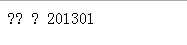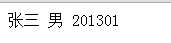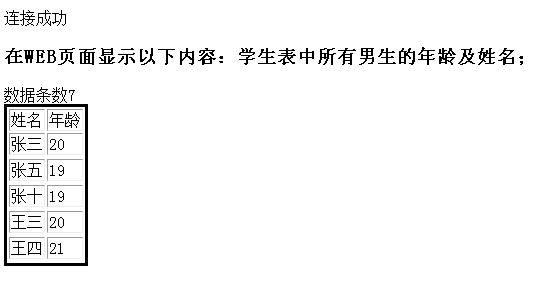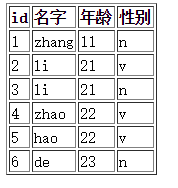• 在php页面显示数据库内容时，中文显示乱码
在php页面中显示数据库内容时，中文不能正确显示，而出现乱码，如下：此时你可尝试加入以下函数语句：
$this->link->query("set names utf8");//其中$this->link是我连接数据库connect（...）时返回的对象
此时就可以正确显示中文了，如下：展开全文• 初次学习用php操作数据库，做一做笔记，简单的总结一下如何web界面输出查询内容和向数据库中添加记录。如有错误，望评论指正。 就是用php嵌套HTML代码，用一个table输出。 学习中遇到一些问题： 1.PHP...
初次学习用php操作数据库，做一做笔记，简单的总结一下如何在web界面输出查询内容和向数据库中添加记录。如有错误，望评论指正。

就是用php嵌套HTML代码，用一个table输出。
在学习中遇到一些问题：

1.PHP无法向MYSQL插入中文记录
如果在sql语句里面插入的字段有中文的话，在web界面会出现乱码的情况
解决方法：最重要的两个步骤就是
1).打开my.ini文件：找到default-character-set，把它设置为utf8,default-character-set=utf8
2).在php代码里面写上mysqli_query("set names 'utf8'");
具体设置看此链接：
点击打开链接
2.每次执行一次insert的sql语句就会向数据库中插入同样的数据
解决方法：设置主键唯一标识
以下是整个代码：

<!doctype html>
<html>
<meta charset="utf-8">
<title></title>
<body>
<table style='text-align:left;border:solid' border="1">
<tr><td>姓名</td><td>年龄</td></tr>
<?php
$conn = mysqli_connect('localhost','root',''); if($conn){
echo '连接成功';
}else{
echo '连接失败';
}

mysqli_query($conn,"insert into myapp.course values('008','计算机系统',48,6,'002')"); mysqli_query($conn, "insert into myapp.course
values('009','嵌入式',48,6,'001')");
mysqli_query($conn,"insert into myapp.student values('98030110','张九',20,'女','980301','03')"); mysqli_query($conn, "insert into myapp.student values('98030111','张十',19,'男','980301','04')");

mysqli_query($conn,"use myapp"); mysqli_query($conn,"set name utf-8");

$sql = 'select Sname,Sage from Student where ssex="男"';$retval = mysqli_query($conn,$sql);
echo '<h3>在WEB页面显示以下内容：学生表中所有男生的年龄及姓名；</h3>';

// $row=mysqli_fetch_assoc($retval);
// print_r($row); //$row=mysqli_fetch_assoc($retval); // print_r($row);//这个只能一行一行的输出

$num=mysqli_num_rows($retval);
//这里用一个for循环输出所有满足条件的查询语句
for ($i=0;$i <$num ;$i++) {
$row=mysqli_fetch_assoc($retval);
$name=$row['Sname'];
$age=$row['Sage'];
echo "<tr><td>$name</td><td>$age</td><tr>";

}
//快速获取数据的条数,不用通过查询所有条数
$result=mysqli_query($conn,'select count(*)from course');
$num1=mysqli_fetch_array($result);
echo '数据条数'.$num1; ?> </table> </body> </html> 这是运行后的效果：展开全文• php查询数据库信息返回到页面中 <?php error_reporting(E_ALL ^ E_NOTICE);$dbhost = 'localhost:3306'; $dbuser = 'root';... php查询数据库信息返回到页面中 改一下$row[‘这里内容即可’]；

<?php
error_reporting( E_ALL ^ E_NOTICE );
$dbhost = 'localhost:3306';$dbuser = 'root';
$dbpass = 'root';$conn = mysqli_connect( $dbhost,$dbuser, $dbpass ); mysqli_query($conn, 'set names utf8' );
mysqli_select_db( $conn, 'management' );$sql = 'select *  FROM  product';
$retval = mysqli_query($conn, $sql ); while($row = mysqli_fetch_array( $retval ) ) { echo "<tr class='tabre'> <td>{$row['id']}</td> ".
"<td>{$row['brand']}</td> ". "<td>{$row['products']} </td> ".
"<td>{$row['original']} </td> ". "<td>{$row['popularity']} </td> ".
"<td><a href='property-one.php?id={$row['id']} ? 新品={$row['listing']}'?>{$row['listing']}</a><br> <a href='property-two.php?id={$row['id']} ? 热卖={$row['cakes']}' ?>{$row['cakes']}</a>
</td> ".
"<td> {$row['recommend']}</td> ". "<td> <a class='compile' href='recommend.php?id={$row['id']}?推荐={$row['recommend']}''>推荐</a>| <a class='compile' href='product-compile.php?id={$row['id']}'>修改</a>|
<a class='delete' href='product-delete.php?id={$row['id']}'>删除</a> </td> ". '</tr>'; } mysqli_close($conn );//关闭
?>

可分开写

<?php
error_reporting( E_ALL ^ E_NOTICE );
$dbhost = 'localhost:3306';$dbuser = 'root';
$dbpass = 'root';$conn = mysqli_connect( $dbhost,$dbuser, $dbpass ); //链接 数据库 mysqli_query($conn, 'set names utf8' );
// 防止乱码
mysqli_select_db( $conn, 'management' );$sql = 'select *  FROM  product';
$retval = mysqli_query($conn, $sql ); ?> 将这个写在最上面开启不要关闭数据库（写在一个文件中才可以取到值） <?php while($row = mysqli_fetch_array( $retval ) ) { echo "<tr class='tabre'> <td>{$row['id']}</td> ".
"<td>{$row['brand']}</td> ". "<td>{$row['products']} </td> ".
"<td>{$row['original']} </td> ". "<td>{$row['popularity']} </td> ".
"<td><a href='property-one.php?id={$row['id']} ? 新品={$row['listing']}'?>{$row['listing']}</a><br> <a href='property-two.php?id={$row['id']} ? 热卖={$row['cakes']}' ?>{$row['cakes']}</a>
</td> ".
"<td> {$row['recommend']}</td> ". "<td> <a class='compile' href='recommend.php?id={$row['id']}?推荐={$row['recommend']}''>推荐</a>| <a class='compile' href='product-compile.php?id={$row['id']}'>修改</a>|
<a class='delete' href='product-delete.php?id={$row['id']}'>删除</a> </td> ". '</tr>'; } mysqli_close($conn );//关闭
?>


展开全文mysql
• 最近由于工作需要把MYSQL里的内容在网页上显示出来，这怎么能实现呢？？ 我也是小白，从网上找了好多源码都不能正常运行，后来把找到的内容整理了一下，大家运行成功就给个赞~~ 首先，先建好自己的数据库并且添加...
最近由于工作需要把MYSQL里的内容在网页上显示出来，这怎么能实现呢？？
我也是小白，从网上找了好多源码都不能正常运行，后来把找到的内容整理了一下，大家运行成功就给个赞~~
首先，先建好自己的数据库并且添加一些的信息在里面，这里我就不说了，大家自己去弄吧。
我就主要说明一下，怎么将建好的数据库中的信息，显示在网页上面。
首先先建立一个文件夹，然后在里面建立两个文件，
一个是连接数据库的配置文件（conn.php）
一个是将数据库中的信息显示在网页上的文件（students.php）
conn.php中的代码如下所示：
<?php
//连接想要连接的数据库，localhost是本地服务器，root为数据库的账号，我的密码为666666
$con = mysql_connect("localhost","root","666666"); //设置字符集，将字符集设置为utf8 的格式，这是大多数的中文都识别的 mysql_query($con,"SET NAMES 'utf8'");
mysql_query($con,"SET CHARACTER SET utf8"); if(!$con){
die(mysql_error());
}
mysql_select_db("login",con); ?>  students.php中的代码如下： <!DOCTYPE html> <html> <body> <table style='text-align:left;' border='1'> <tr><th>id</th><th>名字</th><th>年龄</th><th>性别</th></tr> <?php //引用conn.php文件 require 'conn.php'; //查询数据表中的数据sql = mysql_query("select * from login");
$datarow = mysql_num_rows($sql); //长度
//循环遍历出数据表中的数据
for($i=0;$i<$datarow;$i++){
$sql_arr = mysql_fetch_assoc($sql);
$id =$sql_arr['id'];
$name =$sql_arr['name'];
$age =$sql_arr['age'];
$sex =$sql_arr['sex'];
echo "<tr><td>$id</td><td>$name</td><td>$age</td><td>$sex</td></tr>";
}
?>
</table>
</body>
</html>

运行后的样子就是这样：小白现学现卖，有什么不对的地方大家请指出来，谢谢~~~


展开全文mysql
• /*链接数据库*/ $con = mysql_connect('localhost','root',''); /*链接数据表*/$db_selected = mysql_select_db('users',$con); /*定义编码格式*/ mysql_query('set names utf8');$sql = '...html
• 2.在php中使用 $charset=mysqli_character_set_name($con); //返回数据库默认字符集的编码utf8 echo "默认字符集为: " . $charset; 3.php头部的编码规则应与数据库一致 header("Content-type:text/html... • (https://img-ask.csdn.net/upload/201508/02/1438484186_775697.png) (https://img-ask.csdn.net/upload/201508/02/1438484178_845973.png) (https://img-ask.csdn.net/upload/201508/02/1438484168_708829.png)编码 • 今天我用PHPmyAdmin操作MySQL数据库汉字显示正常，但用PHP网页显示MySQL数据时所有汉字都变成了?号。 原因：没有PHP网页中用代码告诉MySQL该以什么... 2.PHP页面在保存的时候使用utf-8编码保存，可以用记事本或cophpmyadmin • <?php$conn=mysql_connect('127.0.0.1','root',''); mysql_query('use test',$conn); mysql_query('set names utf8',$conn)... //每页显示的记录数 $page=$_GET['page']; //获得当前的页面值 $count=mysql_query("se分页 mysql • 数据库php执行的结果中出现乱码的情况，可能是每个初学者都可能遇到的问题，经过不断的研究和解决，找到了网络上总结的一些不错的解决方法：乱码其实就是因为各个地方编码方式不一样造成的，下面有几个可能...mysql character phpmyadmin • 小弟现在开始学习MVC，现在用database first的方法去实施，连接上了本地数据库之后，要怎么写控制器和上下文类来让数据在页面显示呢？mvc 数据 方法 • 3.并且把数据库查询得到的内容填写到跳转网页的表单中 【说一下思路】 1.点击按钮之后，触发函数，这个函数能够获取到页面中被选中要修改的项目的标识，通过ajax将此值传给php文件。 2.接下来php会进行... • ...<?php //一个东西搞定 str_replace("\n"," ",$str); ?> 厉害, 今天看php发现有一个可以自动换行的函数,更加简单 nl2br($str); 这个函数可以把 字符串里面的 \n 转化为 • //设置页面字符集 header("content-type:text/html;charset=utf-8"); //链接数据库$con=mysql_connect("localhost","root","root"); //判断数据库连接是否成功 if(!$con){ die(mysql_error()); }else{ ... • 把表单的数据成对存到数组的键和值中，然后json化存到数据库在取出来反转显示页面中，保持键和值对 HTML form表单 input name="key[]" id="name" value="0"> 键 input name="key[]" id="name" ...json 表单 • 其实很简单的，把需要转换的数据 提取出来 例：$cartdata = htmlspecialchars_decode($data['content']); 输出$cartdata 即可html
• // 不操作数据库，直接填充数据显示 echo $form->field($model, 'edu')->dropDownList(['1'=>'大学','2'=>'高中','3'=>'初中'], ['prompt'=>'请选择','style'=>'width:120px']) ?> // 下拉框读取数据库操作 ...
• ## PHP中将数据库中的数据显示在网页

万次阅读 多人点赞 2015-12-16 11:45:24
这次讲的是mysql数据库中的数据使用php如何显示在网页中。首先，先建好自己的数据库并且添加一些的信息里面，如果大家再看这篇文章的话，相信mysql还是懂一些的，建库就是使用一些的create之类的了，这里我就不说...
• 很多博客软件都有这么一个功能，比如“生成本次页面一共花费了xx毫秒，进行了xx次数据库查询”等等。那么这个功能是如何实现的呢，下面我大概说下思路。
• 数据库读取图片路径后在页面显示出来 代码: 1 //直接将代码放到php文件里 2 $con = mysqli_connect("localhost", "123", "123", "123");//连接数据库 3$sql = "SELECT * FROM table";//读取表 4 $result... • <?php /* *菜鸟分享 如果代码有错误,忘指点....$link = mysql_connect("localhost","root","root")or die('数据库连接失败!原因:'.mysql_error()); mysql_select_db("bygd", $link); mysql_set_charset • 这次是一个CI的实例，功能为通过CI框架，在页面显示数据库中的内容mvc 框架 • 着是困惑很多人的问题，如果处理不好，都是乱码，说这些话并不是我对编码很精通，只是这方面是得留神，自己总结了一点小经验(容易出现乱码的地方有php文件里面 ，数据库里面 存储 的编码 ，页面显示 ...encoding phpmyadmin myeclipse • 首先显示一下数据库的数据 页面信息 http://www.w3.org/TR/xhtml1/DTD/xhtml1-transitional.dtd"> http://www.w3.org/1999/xhtml"> 无标题文档 留言内容 编号用户标题内容发表时间$link=...
• /***************************by garcon1986************************************/<?phperror_reporting(E_ALL ^ E_NOTICE);//create database test//create table php_radio(id int(10) NOT NULL AUTO_INCquery input mysql
• 现在的问题是这样的：我想上传图片，并将图片的路径传到数据库，为了另外一个页面显示图片的地方能够获取到数据库内容。select下拉框有几个值，想提交到数据库好像有点bug用正常的$_POST["select的name"] 似乎...图片 表单 • 这个是对上次博文修改和完善，...mysql_connect.php 数据库连接页面 ms_login.php数据库中提取数据验证 register.php 注册页面 register_1.php 是注册验证，并写入到数据库中 showing.php 图片验证码 huangyinmysql input class • 数据库中有数据表hacker，现在我们要将hacker表中的数据查询出来并在页面显示，代码如下： <?php // 连接数据库$mysqli = mysqli_connect("localhost","root","123",'student'); //mysqli_close($con);$...mysql sql...